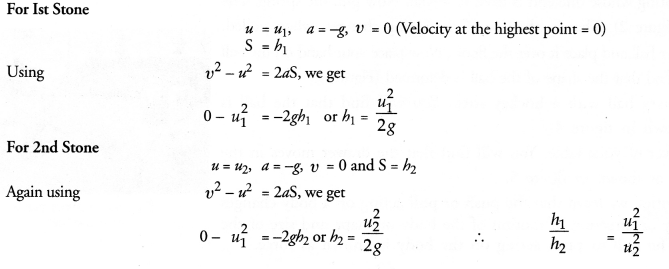## NCERT Exemplar Solutions for Class 9 Science Chapter 8 Motion

These Solutions are part of NCERT Exemplar Solutions for Class 9 Science . Here we have given NCERT Exemplar Solutions for Class 9 Science Chapter 8 Motion

MULTIPLE CHOICE QUESTIONS

Question 1.
A particle is moving in a circular path of radius r. The displacement after half a circle would be :
(a) Zero       (b) πr            (c) 2r                  (d) 2πr.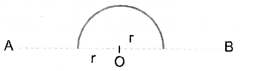(c) Explanation : Particle is just opposite to the initial position on the circle.

More Resources

Question 2.
A body is thrown vertically upward with velocity u, the greatest height h to which it will rise is,
(a) u/g             (b) u2/2g                (c) u2/g                (d) u/2g.
(b) Explanation : u2 – v2 = – 2gh or 0 – u2 = – 2gh (∴ u = 0 at highest point).
∴ h = u2/2g.

Question 3.
The numerical ratio of displacement to distance for a moving object is
(a) always less than 1
(b) always equal to 1
(c) always more than 1
(d) equal or less than 1
(d) Explanation : Displacement ≤ distance.

Question 4.
If the displacement of an object is proportional to square of time, then the object moves with
(a) uniform velocity
(b) uniform acceleration
(c) increasing acceleration
(d) decreasing acceleration.Question 5.
From the given u -t graph (Fig. 1), it can be inferred that the object is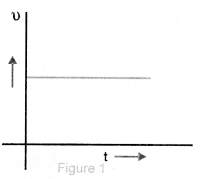(a) in uniform motion
(b) at rest
(c) in non-uniform motion
(d) moving with uniform acceleration.
(a) Explanation : Velocity of object is constant.

Question 6.
Suppose a boy is enjoying a ride on a merry-go-round which is moving with a constant speed of 10 m s-1. It implies that the boy is
(a) at rest
(b) moving with no acceleration
(c) in accelerated motion
(d) moving with uniform velocity.
(c) Explanation : Velocity of the boy changes continuously due to the change in direction of motion in circular path.

Question 7.
Area under a v — t graph represents a physical quantity which has the unit
(a) m2          (b) m                (c) m3              (d) m s-1.
(b) Explanation : Area = v x t = (m/s) x s = m.

Question 8.
Four cars A, B, C and D are moving on a levelled road. Their distance versus time graphs are shown in Fig. 2 Choose the correct statement Q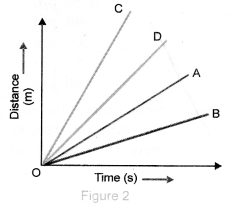(a) Car A is faster than car D
(b) Car B is the slowest
(c) Car D is faster than car C
(d) Car C is the slowest.
(b) Explanation : Slope of distance – time graph = speed of object.

Question 9.
Which of the following figures represents uniform motion of a moving object correctly ?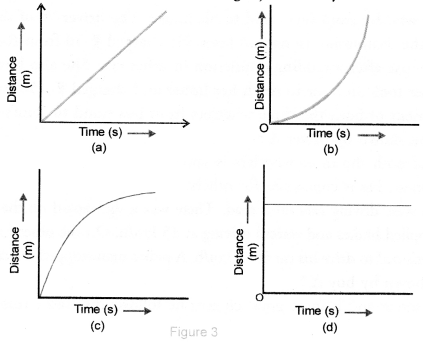(a) Explanation : For uniform motion, distance ∞ time.

Question 10.
Slope of a velocity – time graph gives
(a) the distance
(b) the displacement
(c) the acceleration
(d) the speed.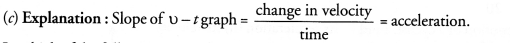Question 11.
In which of the following cases of motions, the distance moved and the magnitude of displacement are equal ?
(a) If the car is moving on straight road
(b) If the car is moving in circular path
(c) The pendulum is moving to and fro
(d) The earth is revolving around the Sun.
(a)

Question 12.
The displacement of a moving object in a given interval of time is zero. Would the distance travelled by the object also be zero ? Justify your answer.
No. When object moves in a circular path of radius r, then displacement of object .after completing a circle is zero but distance travelled = 2πr.

Question 13.
How will the equations of motion for an object moving with a uniform velocity change ?
Equations of motion of a uniformly accelerated motion of an object are

1. V = u + at,
2. S = ut + ½ at2,
3. v2 – u2 = 2aS.

When object moves with a uniform velocity, its acceleration, a = 0. Hence equations of motion become

1. V = u,
2. S = ut,
3. v= u2.

Question 14.
A girl walks along a straight path to drop a letter in the letterbox and comes back to her initial position. Her displacement-time graph is shown in Fig. 4. Plot a velocity-time graph for the same.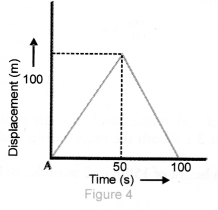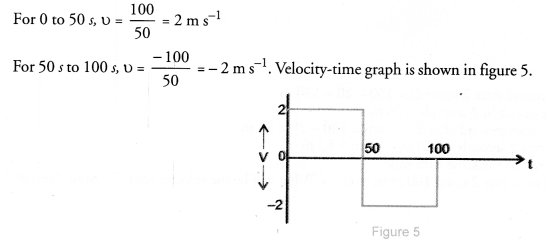Question 15.
A car starts from rest and moves along the x-axis with constant acceleration 5 m s-2 for 8 seconds. If it then continues with constant velocity, what distance will the car cover in 12 seconds since it started from the rest ?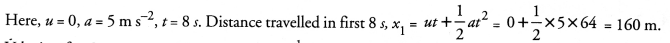Velocity after 8 s,V = u + at = 0 + 5 x 8 = 40 ms-1.
Distance travelled in last 4 s moving with constant velocity, x2 = v t = 40 x 4 = 160 m
∴Total distance = x1 + x2 = 320 m.

Question 16.
A motorcyclist drives from A to B with a uniform speed of 30 km h-1 and returns back with a speed of 20 km h-1. Find its average speed.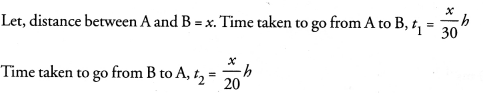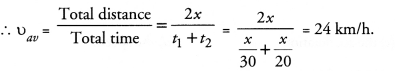Question 17.
The velocity-time graph (Fig. 6) shows the motion of a cyclist. Find
(i) its acceleration
(ii) its velocity and
(iii) the distance covered by the cyclist in 15 seconds.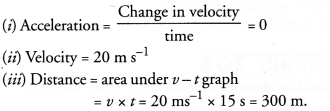Question 18.
Draw a velocity versus time graph of a stone thrown vertically upwards and then coming downwards after attaining the maximum height
When stone is thrown vertically upwards, it has some initial velocity (u). The velocity of the stone goes on decreasing as it goes upwards and becomes zero at the maximum height. There after, stone begins to fall and its velocity goes on increasing but in opposite direction and becomes equal to the initial velocity (u) when it reaches the point of projection. v – t graph of a stone is shown in figure 7 A. The speed-time graph of the stone is shown in figure 7 B.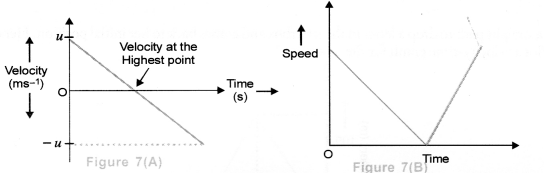Question 19.
An object is dropped from rest at the height of 150m and simultaneously another object is dropped from rest at the height of 100m.
What is the difference in their heights after 2 s if both the objects drop with same accelerations ? How does the difference in heights vary with time ?
Distance moved by the object dropped from height of 150m in 2 seconds can be calculated using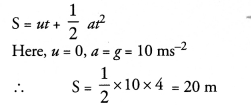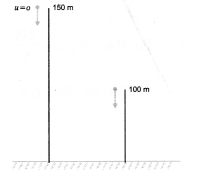Height of the object from ground after 2 seconds = 150 – 20 = 130 m Similarly, distance moved by the other object in 2 seconds = 20 m
Height of the second object from ground after 2 seconds = 100 – 20 = 80 m
∴ Difference in their heights after 2 seconds = 130 m – 80 m = 50 m
Difference in height of both objects remains the same with time.

Question 20.
An object starting from rest travels 20 m in first 2 s and 160 m in next 4 s. What will be the velocity after 7 s from the start ?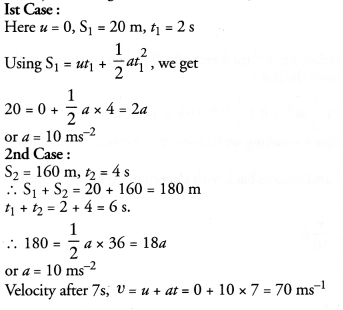Question 21.
Using following data, draw time-displacement graph for a moving object :

 Time (s)     0 2 4 6 8 10 12 14 16 Displacement (m) 0 2 4 4 4 6 4 2 0

Use this graph to find average velocity for first 4 s, for next 4 s and for last 6 s.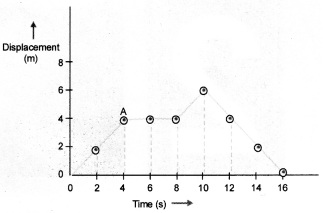Displacement-time graph is shown in figure 8.
Average velocity for first 4s = Slope of displacement-time graphQuestion 22.
An electron moving with a velocity of 5 x 104 ms-1 enters into a uniform electric field and acquires a uniform acceleration of 104 ms-2 in the direction of its initial motion.
(i) Calculate the time in which the electron would acquire a velocity double of its initial velocity.
(ii) How much distance the electron would cover in this time ?Question 23.
Obtain a relation for the distance travelled by an object moving with a uniform acceleration in the interval between 4th and 5th seconds.